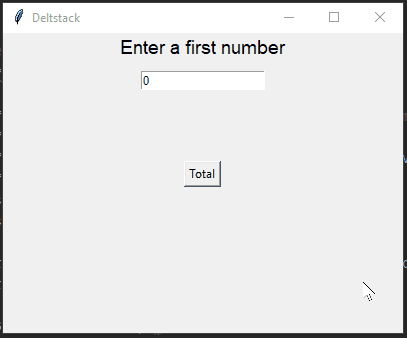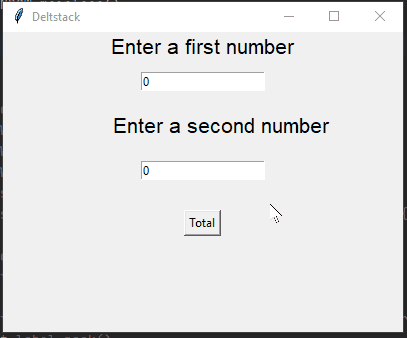# Tkinter Intvar

Tkinter 包含各种内置程序，它们的行为类似于标准 Python 数据类型，具有用于非常有效地欺骗 Tkinter 的组件（如标签和条目组件）的附加功能，使其与 Python 数据类型不同。

## 在 Tkinter 中使用 `IntVar()` 创建一个整数变量

``````from tkinter import *
``````

``````first_intvar=IntVar()
``````

``````def showDatatype():
second_label.config(text=f'{first_intvar.get()}{type(first_intvar.get())}')
``````

``````from tkinter import *
# Create GUI window object of TK
GUI_WINDOW=Tk()
GUI_WINDOW.title("Deltstack")
GUI_WINDOW.geometry("400x300")
def showDatatype():
second_label.config(text=f'{first_intvar.get()}{type(first_intvar.get())}')
# Create integer object using IntVar class
first_intvar=IntVar()
first_label=Label(GUI_WINDOW,text="Enter a first number",font=("bold 14"))
first_label.pack()
first_entry=Entry(GUI_WINDOW,textvariable=first_intvar)
second_label=Label(GUI_WINDOW,text="")
total_btn=Button(GUI_WINDOW,text="Total",command=showDatatype)
GUI_WINDOW.mainloop()
``````## 在 Tkinter 中使用 `IntVar()` 计算两个数字的和

``````first_intvar=IntVar()
second_intvar=IntVar()
``````

``````answer=Label(GUI_WINDOW,text="",font=("itelic 13"))
``````

``````def sumTwoNumbers():
try:
# Try to getting integer values and sum two numbers
total=first_intvar.get()+second_intvar.get()
answer.config(text=f"The sum of two numbers is {total}")
except Exception:
``````

``````from tkinter import *
# Create GUI window object of TK
GUI_WINDOW=Tk()
GUI_WINDOW.title("Deltstack")
GUI_WINDOW.geometry("400x300")
def sumTwoNumbers():
try:
# Try to getting integer values and sum two numbers
total=first_intvar.get()+second_intvar.get()
answer.config(text=f"The sum of two numbers is {total}")
except Exception:
# Create integer object using IntVar class
first_intvar=IntVar()
second_intvar=IntVar()
first_label=Label(GUI_WINDOW,text="Enter a first number",font=("bold 16"))
first_label.pack()
first_entry=Entry(GUI_WINDOW,textvariable=first_intvar)
second_label=Label(GUI_WINDOW,text="      Enter a second number",font=("bold 16"))